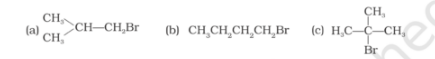# Arrange the following compoundsQuestion:

Arrange the following compounds in increasing order of their boiling points.(i) (b) < (a) < (c)

(ii) (a) < (b) < (c)

(iii) (c) < (a) < (b)

(iv) (c) < (b) < (a)

Solution:

Option (iii)  (c) < (a) < (b) is the answer.